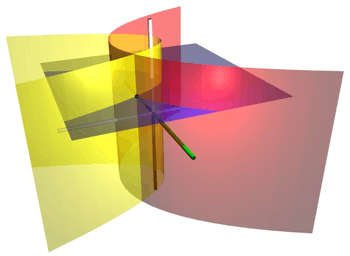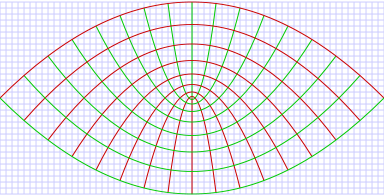# Parabolic cylindrical coordinatesCoordinate surfaces of parabolic cylindrical coordinates. The red parabolic cylinder corresponds to σ=2, whereas the yellow parabolic cylinder corresponds to τ=1. The blue plane corresponds to z=2. These surfaces intersect at the point P (shown as a black sphere), which has Cartesian coordinates roughly (2, -1.5, 2).

In mathematics, parabolic cylindrical coordinates are a three-dimensional orthogonal coordinate system that results from projecting the two-dimensional parabolic coordinate system in the perpendicular-direction. Hence, the coordinate surfaces are confocal parabolic cylinders. Parabolic cylindrical coordinates have found many applications, e.g., the potential theory of edges.

## Basic definitionParabolic coordinate system showing curves of constant σ and τ the horizontal and vertical axes are the x and y coordinates respectively. These coordinates are projected along the z-axis, and so this diagram will hold for any value of the z coordinate.

The parabolic cylindrical coordinates (σ, τ, z) are defined in terms of the Cartesian coordinates (x, y, z) by:The surfaces of constant σ form confocal parabolic cylindersthat open towards +y, whereas the surfaces of constant τ form confocal parabolic cylindersthat open in the opposite direction, i.e., towards y. The foci of all these parabolic cylinders are located along the line defined by x = y = 0. The radius r has a simple formula as wellthat proves useful in solving the Hamilton-Jacobi equation in parabolic coordinates for the inverse-square central force problem of mechanics; for further details, see the Laplace–Runge–Lenz vector article.

## Scale factors

The scale factors for the parabolic cylindrical coordinates σ and τ are:## Differential elements

The infinitesimal element of volume isThe differential displacement is given by:The differential normal area is given by:## Del

Let f be a scalar field. The gradient is given byThe Laplacian is given byLet A be a vector field of the form:The divergence is given byThe curl is given byOther differential operators can be expressed in the coordinates (σ, τ) by substituting the scale factors into the general formulae found in orthogonal coordinates.

## Relationship to other coordinate systems

Relationship to cylindrical coordinates (ρ, φ, z):Parabolic unit vectors expressed in terms of Cartesian unit vectors:## Parabolic cylinder harmonics

Since all of the surfaces of constant σ, τ and z are conicoids, Laplace's equation is separable in parabolic cylindrical coordinates. Using the technique of the separation of variables, a separated solution to Laplace's equation may be written:and Laplace's equation, divided by V, is written:Since the Z equation is separate from the rest, we may writewhere m is constant. Z(z) has the solution:Substituting m2 for, Laplace's equation may now be written:We may now separate the S and T functions and introduce another constant n2 to obtain:The solutions to these equations are the parabolic cylinder functionsThe parabolic cylinder harmonics for (m, n) are now the product of the solutions. The combination will reduce the number of constants and the general solution to Laplace's equation may be written:## Applications

The classic applications of parabolic cylindrical coordinates are in solving partial differential equations, e.g., Laplace's equation or the Helmholtz equation, for which such coordinates allow a separation of variables. A typical example would be the electric field surrounding a flat semi-infinite conducting plate.